##### Signals and Systems For Dummies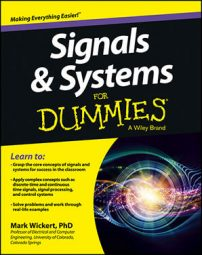Here are eleven common mistakes students make when trying to solve problems and how to avoid them. Slow down enough to think through solutions, and make sure your fundamental understanding of the core material is at least as good as your ability to work through detailed problems.

## Miscalculating the folding frequency

In sampling theory, the alias frequencies fold over fs / 2 (known as the folding frequency), where fs is the sampling frequency in hertz. An error in the calculation of the principle alias or the alias frequency results when you use the folding frequency improperly.

Consider fs = 10 Hz and the calculation of the principle alias frequency relative to f = 7 Hz. You may quickly reason that the principle alias frequency is 7 – fs / 2 = 7 – 5 = 2 Hz, because 7 folds about 5 to produce 7.

This is wrong! This isn’t the folding frequency interpretation. Because 7 Hz is 2 Hz above 5 Hz, the corresponding folded frequency is 2 Hz below 5 Hz, or 3 Hz.

This same concept can be misunderstood when you’re given a principle alias frequency and need to find the alias frequency on the interval [fs / 2, fs]. With 10 Hz, suppose the principle alias is f0 = 4 Hz.

The nearest alias frequency isn’t fs / 2 + 4 = 5 + 4 = 9 Hz; the principle alias sits 1 Hz below the folding frequency so the corresponding alias frequency is 1 Hz above the folding frequency or 6 Hz.

In a causal system, only the present and past values of the input can form the present output. When given a system input/output relationship, such as y(t) = 5x(t – 2) + u(t + 5), don’t be thrown off by the u(t + 5). The system is causal because the input two seconds in the past forms the present value of the output.

The system also contains a time-varying bias that turns on at t = –5. This bias is part of the system and isn’t related to the input x(t).

## Plotting errors in sinusoid amplitude spectra

Plotting the two-sided amplitude spectra of sinusoidal signals seems so easy, but students too frequently ignore or forget about the 1/2-amplitude scaling factor from Euler’s formula.

Consider a signal composed of a single sinusoid and a direct current component (DC):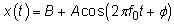Create the two-sided line spectra by expanding the cosine and using Euler’s formula: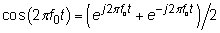Applying the expansion to x(t), you get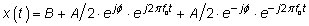There’s a spectral line of amplitude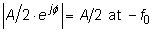due to the positive frequency complex sinusoid, a spectral line of amplitude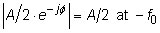due to the negative frequency complex sinusoid, and a spectral line of amplitude |B| (absolute value in case the DC component is negative) at 0 Hz (DC). Did you notice the 2 in A/2 for the spectral lines at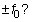Stumbling with angle calculations on basic scientific calculators is an easy mistake to make.

For instance, to find the angle of complex number z = x + jy, maybe you start by finding (y/x), but you need to make note of which quadrant of the complex plane the number is actually in. For Quadrants I and IV, arctan faithfully returns the correct angle.

For a Quadrant II complex number, arctan thinks you’re in Quadrant IV, so you need to add the following to the arctan result: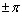For a Quadrant III complex number, arctan thinks you’re in Quadrant I, so you must add the following to the arctan result:The positive or negative is your choice depending on how you like your angle.

## Being unfamiliar with calculator functions

When manipulating complex numbers on your calculator, avoid making careless mistakes:

• Be aware of the angle mode you’ve set for your device. Use radians mode for all your angle calculations, and be consistent. If you need a final answer in degrees, do that at the end by multiplying by 180/π.

• Know how to use your calculator. You may be tempted to borrow a friend’s super calculator but fail to spend any time using it until you’re under the pressure of a quiz or exam.

When you want to find the linear constant coefficient (LCC) difference or differential equation starting from the system function, you may end up swapping the numerator and denominator polynomials by being careless.

The case in point here is for the z-domain. Say you’re given the following equation and asked to find the difference equation from H(z):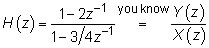You notice Y(z) across from 1 – 2z–1 and X(z) across from 1 – 3 / 4z–1 and may think y[n] – 2y[n – 1] = x[n] – 3 / 4x[n – 1]. But that approach is wrong. To get back to the difference equation, you need to cross-multiply: Y(z) * (1 – 3 / 4z–1) = X(z) * (1 – 2z–1). And then you can correctly write y[n] – 3 / 4y[n – 1] = x[n] – 2x[n – 1].

## Ignoring the convolution output interval

When convolving two functions or two sequences, you need to consider a lot of details. On the heels of forgetting to slow down and take a deep breath, many people forget to first find the convolution output interval from the input signals/sequences x1 and x2.

This simple calculation tells you where you’re going with your final answer. Without it, you can still get a nice answer, but the support interval may be wrong due to other errors.

Given that x1(t) has support interval [t1,t2] and x2(t) has support interval [t3,t4], the convolution y(t) = x1(t) * x2(t) has support interval no greater than [t1 + t3, t2 + t4]. Similar results hold for sequences with t replaced by n.

## Forgetting to reduce the numerator order before partial fractions

When working with inverse Laplace transforms (ILTs) and inverse z-transforms, you typically deal with a rational function, such as N(s) / D(s) or N(z) / D(z). Before you can begin your partial fraction expansion, make sure the function is proper rational.

The surprise with making this careless error is that you’ll get an answer, and you may leave the exam feeling good — until your buddy comments on the need for long division on a problem.

## Forgetting about poles and zeros from H(z)

When finding the poles and zeros of a finite impulse response (FIR) filter for a problem such as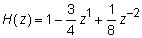forgetting about the two poles at z = 0 is easy. If you just factor the polynomial as (1 – 0.25z–1)(1 – 0.5z–1) and plot zeros at z = 0.25 and z = 0.5, then your solution is wrong.

Find the poles z = 0 by switching to positive powers of z: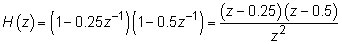There, the poles are now visible. The number of poles and zeros is always equal, but some may be at infinity.

## Missing time delay theorems

When applying the time delay theorem in the Fourier domain, the time shift theorems apply everywhere that the independent variable occurs. Too often, students apply the theorem partially, so some t or n values are left unmodified. For z–3 / (1 – 0.5z–1), the inverse z-transform is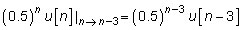Notice the time shift in two places!

## Disregarding the action of the unit step in convolution

In both the continuous- and discrete-time convolution, you may need to flip and slide a signal containing a unit step function. The error occurs when you don’t carefully consider the action of the unit step function with respect to the integration or sum variable.

You may ignore the fact that the flipped and shifted unit step function turns off at some point, rather than on, as the integration or sum index variable increases. Your integration or sum limits likely depend on the turning off behavior, so the problem solution drives off course with one or more errors.